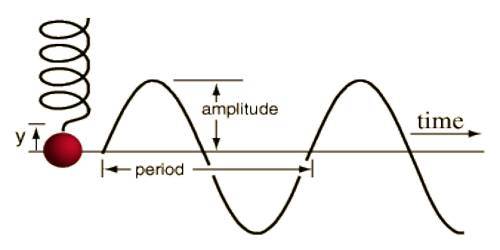# Experimental Explanation of Simple Harmonic Motion

It acceleration of a body executing periodic motion acts along a fixed point in its path of motion in such a way that its magnitude from that point is proportional to its displacement, then that motion of the body is called simple harmonic motion. Motion of a simple pendulum having a small amplitude, vibration of arms of a tuning fork, vertical oscillation of a spring etc.

In figure motion of a particle N vibrating along a straight line between points C and D has been shown. Suppose O is the equilibrium position of the particle and at any moment its displacement from equilibrium is x. If the motion of the particle is simple harmonic motion, then at that moment acceleration a of the particle is proportional to x and it points towards N. That means,

a ∞ – x

or, a = – k x

Here k is proportionality constant. From negative sign it is understood that acceleration is always in the opposite direction of displacement, i.e., acts towards the equilibrium position.Again according to the Newton’s second Law of motion, considering the mass of the particle it can be shown that acceleration is proportional to displacement and is oppositely directed. In our daily life, there are innumerable examples like this, for example motion of the pendulum of a wall clock, oscillation a produced by expanding a spring and then released, all these motions are simple harmonic motion.

Experiment:

Any system that repeats its motion to and fro its mean or rest point  executes simple harmonic motion.

EXAMPLES:

• simple pendulum
• mass spring system
• a steel ruler clamped to a bench oscillates when its free end is displaced sideways.
• a steel ball rolling in a curved dish
• a swing

Thus to get S.H.M a body is displaced away from its rest position and then released. The body oscillates due to restoring force. Under the action of this restoring force the body accelerates and overshoots the rest position due to inertia. The restoring force than pulls it back.

The restoring force is always directed towards the mean position and so the acceleration is also directed towards the mean or rest position.